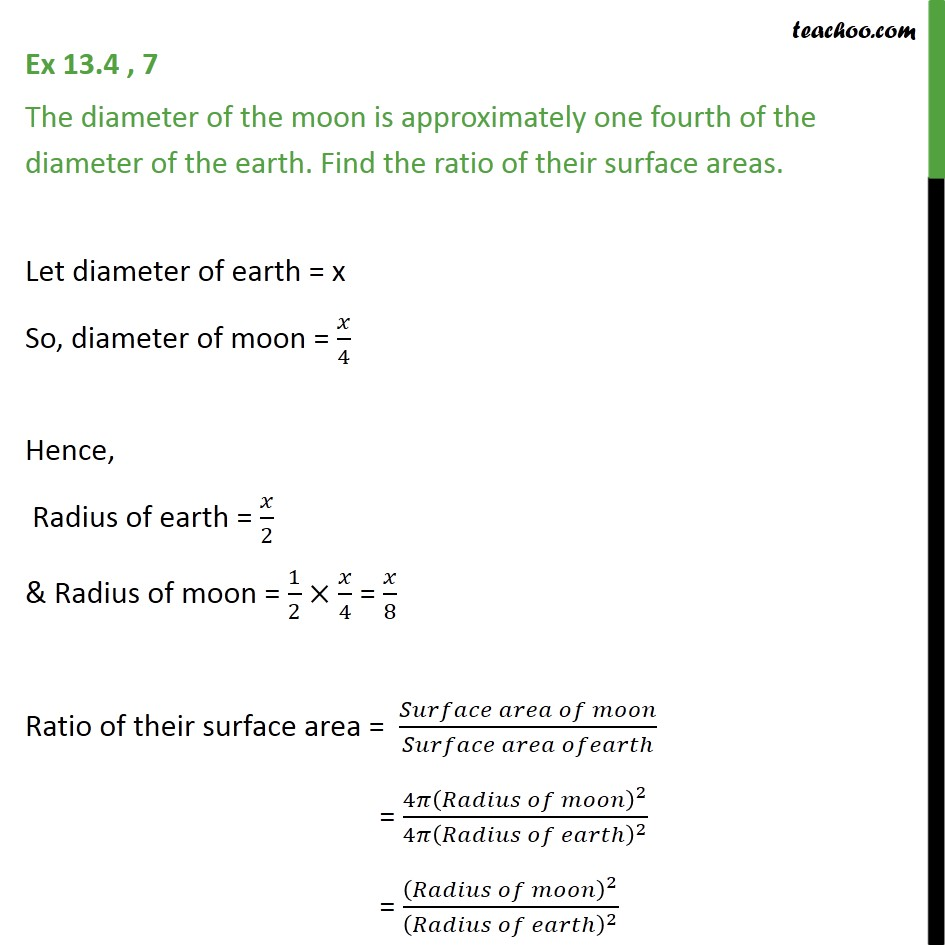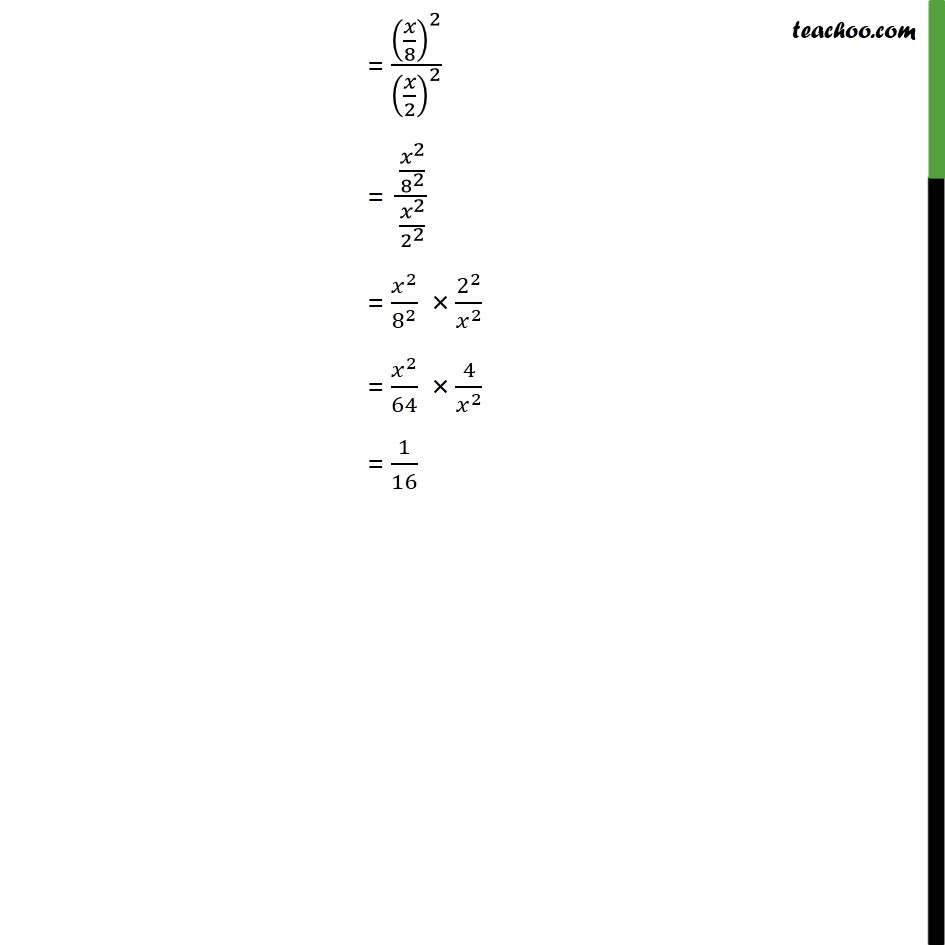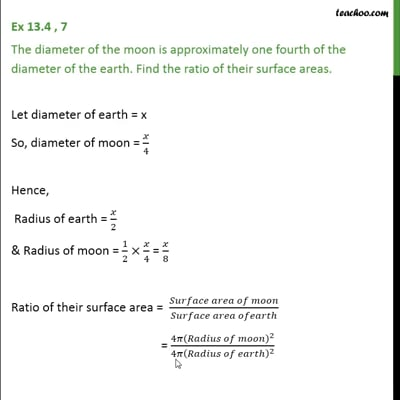Ex 13.4

Chapter 13 Class 9 Surface Areas and Volumes
Serial order wiseThis video is only available for Teachoo black users

Solve all your doubts with Teachoo Black (new monthly pack available now!)

### Transcript

Ex 13.4 , 7 The diameter of the moon is approximately one fourth of the diameter of the earth. Find the ratio of their surface areas. Let diameter of earth = x So, diameter of moon = 𝑥/4 Hence, Radius of earth = 𝑥/2 & Radius of moon = 1/2×𝑥/4 = 𝑥/8 Ratio of their surface area = (𝑆𝑢𝑟𝑓𝑎𝑐𝑒 𝑎𝑟𝑒𝑎 𝑜𝑓 𝑚𝑜𝑜𝑛)/(𝑆𝑢𝑟𝑓𝑎𝑐𝑒 𝑎𝑟𝑒𝑎 𝑜𝑓𝑒𝑎𝑟𝑡ℎ) = (4𝜋(𝑅𝑎𝑑𝑖𝑢𝑠 𝑜𝑓 𝑚𝑜𝑜𝑛)^2)/(4𝜋(𝑅𝑎𝑑𝑖𝑢𝑠 𝑜𝑓 𝑒𝑎𝑟𝑡ℎ)^2 ) = (𝑅𝑎𝑑𝑖𝑢𝑠 𝑜𝑓 𝑚𝑜𝑜𝑛)^2/(𝑅𝑎𝑑𝑖𝑢𝑠 𝑜𝑓 𝑒𝑎𝑟𝑡ℎ)^2 = (𝑥/8)^2/(𝑥/2)^2 = (𝑥^2/8^2 )/(𝑥^2/2^2 ) = 𝑥^2/8^2 × 2^2/𝑥^2 = 𝑥^2/64 × 4/𝑥^2 = 1/16